## Bias

### Wikipedia definition

Defined as: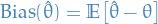1

where the expectation is taken over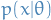, i.e. averaging over all possible observations.

Here we have assumed that the real model follows the same model as we want to use as an estimator, and then we're looking at how the exected value of our estimated parameter for this assumed model differs from the real parameter for the assumed model.

### ESL definition

From The Elements of Statistical Learning, we have the following definition: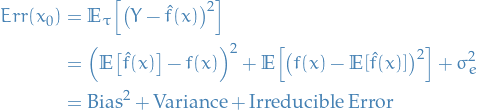2

where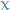is a single observation, therefore we interpret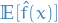as creating the same model over and over, and then taking the expectation of the predictions of all these models, basically like we do in bagging (Bootstrap Aggregation).

Notice how this differs from the Wikipedia definition where we assume that the estimator follows the same model as the real model, but simply using (potentially) different parameters.